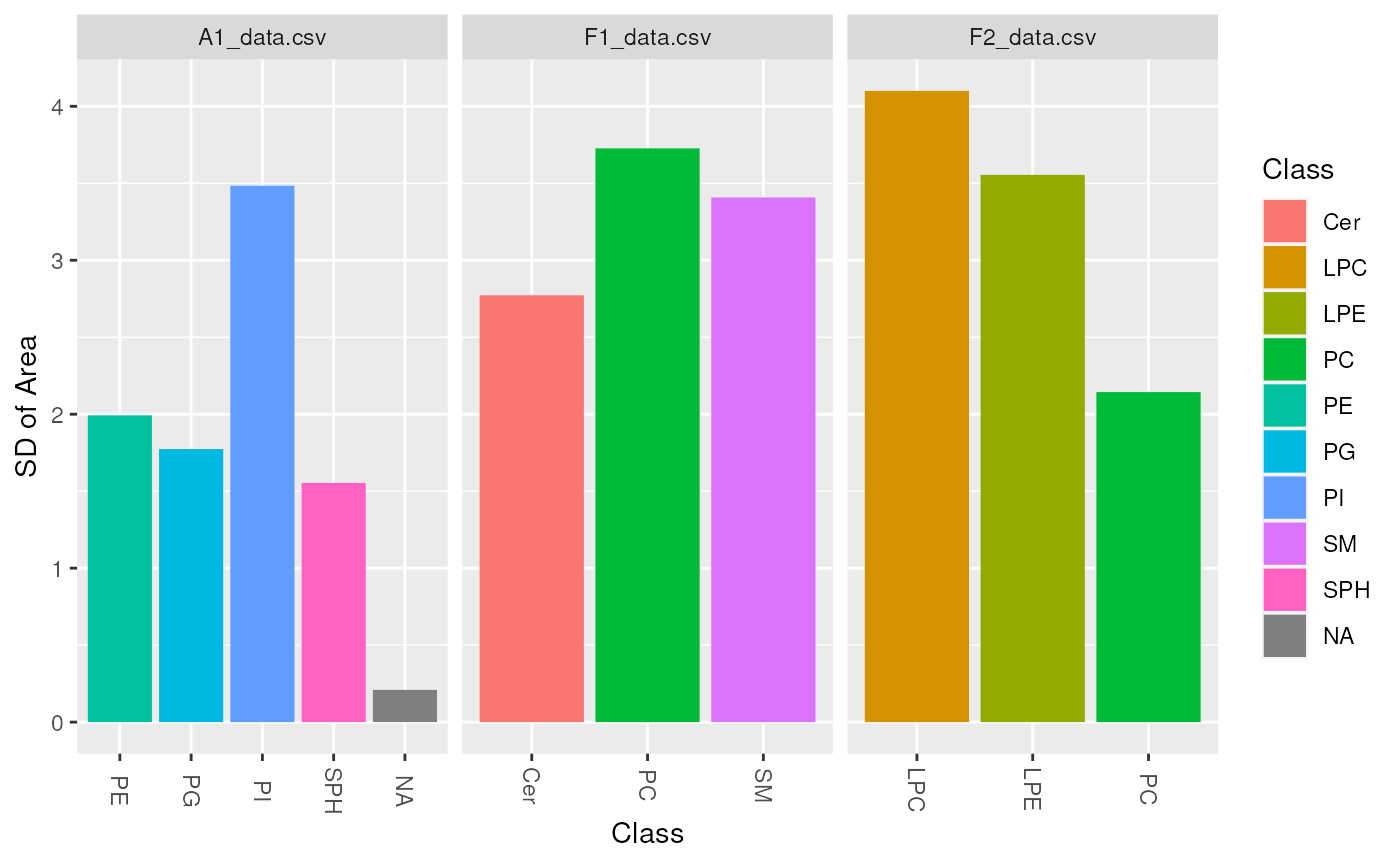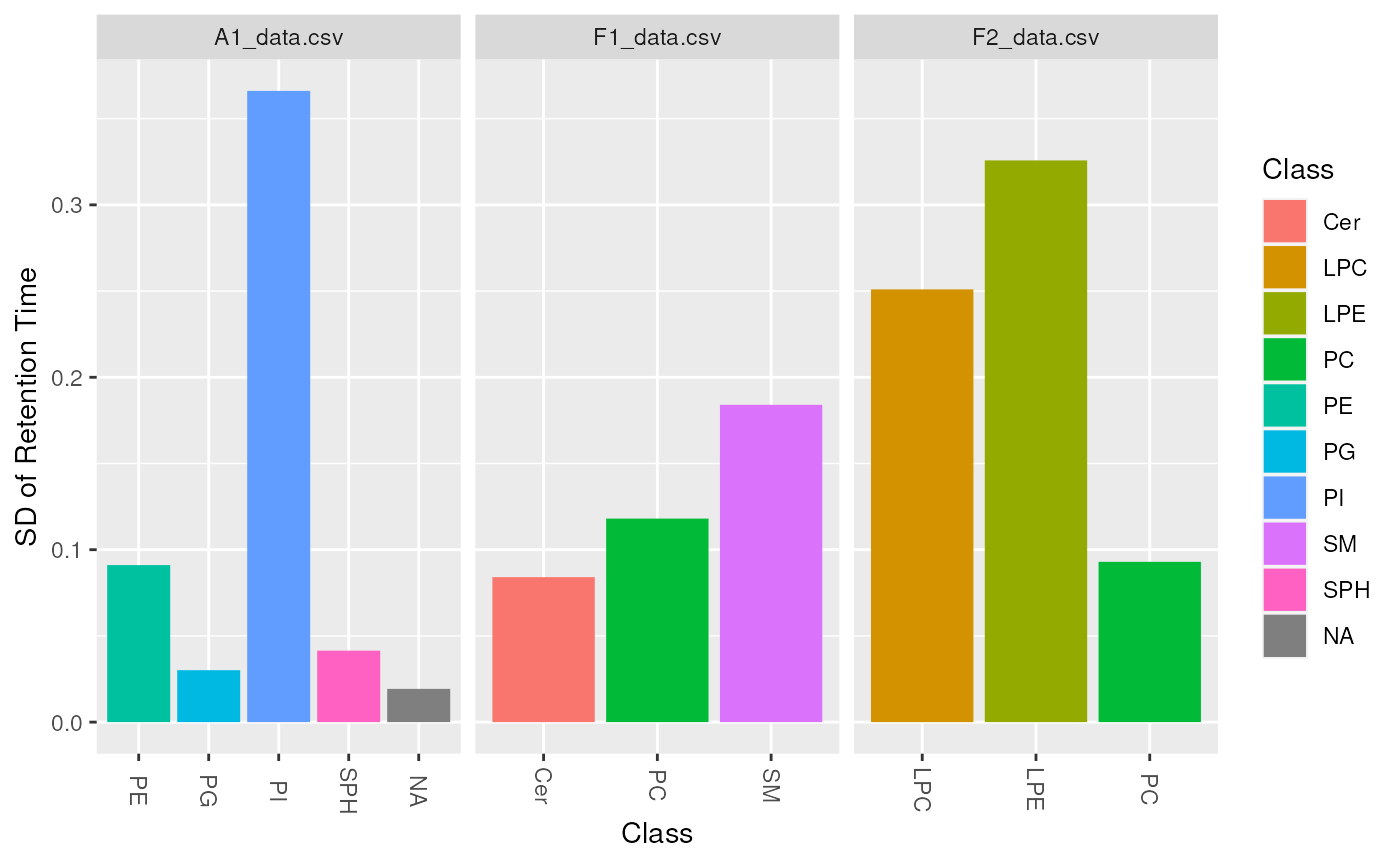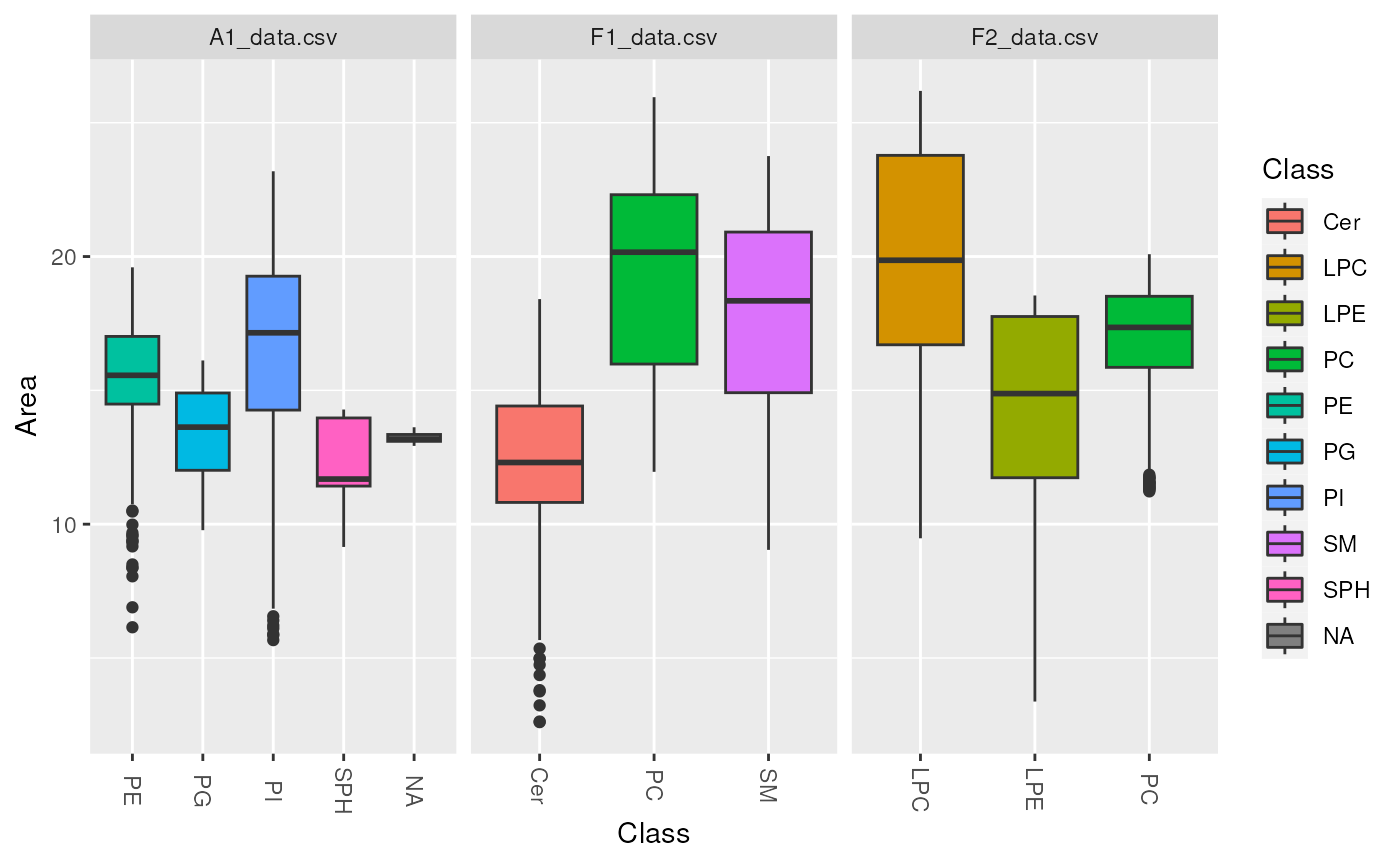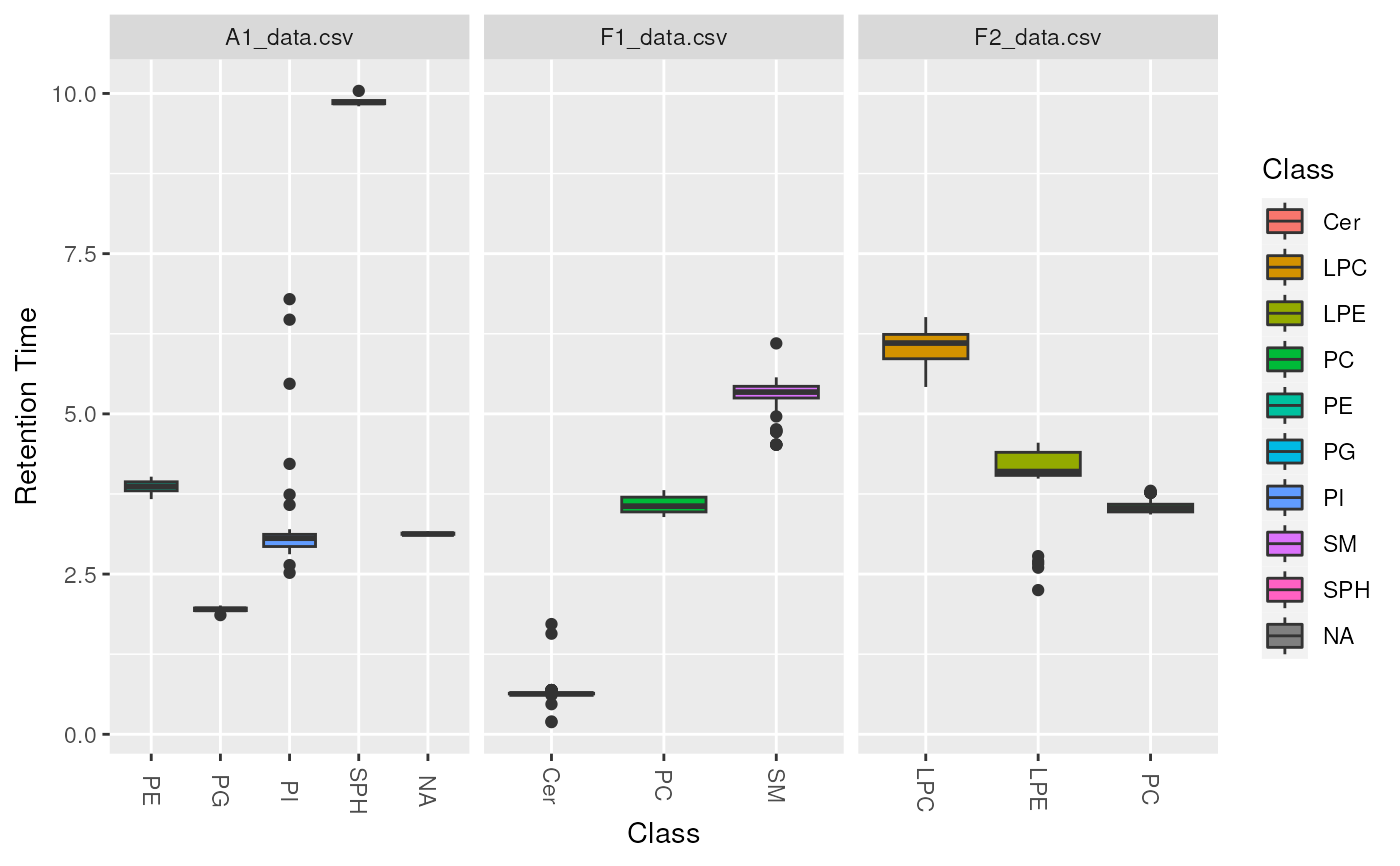lipidr supports two types of plots for to visualize at lipid classes.

sd plots a bar chart for standard deviation of a certain measure in each class. This plot type is usually used to look at standard deviations of intensity in each class, but can also be used to look at different measures such as Retention Time, to ensure all lipids are eluted within the expected range. To assess instrumental variation apply the function to technical quality control samples.

boxplot Plots a boxplot chart to examine the distribution of values per class. This plot type is usually used to look at the intensity distribution in each class, but can also be used to look at different measures, such as Retention Time or Background.

plot_lipidclass(data, type = c("boxplot", "sd"), measure = "Area", log = TRUE)

## Arguments

data

LipidomicsExperiment object.

type

plot type, either boxplot or sd. Default is boxplot.

measure

Which measure to plot the distribution of: usually Area, Area Normalized, Height or Retention Time. Default is Area

log

Whether values should be log2 transformed. Default is TRUE (Set FALSE for retention time).

A ggplot object.

## Examples

data(data_normalized)

d_qc <- data_normalized[, data_normalized\$group == "QC"]
plot_lipidclass(d_qc, "sd", "Area", log = TRUE)plot_lipidclass(d_qc, "sd", "Retention Time", log = FALSE)
#> Warning: Removed 1 rows containing non-finite values (stat_summary).plot_lipidclass(d_qc, "boxplot", "Area", log = TRUE)plot_lipidclass(d_qc, "boxplot", "Retention Time", log = FALSE)
#> Warning: Removed 1 rows containing non-finite values (stat_boxplot).